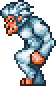\l!--\_\n --\g\{\{#lstmap:\$\$\$\!+\!@@@@\!\l!--\n --\g\{\\{#if:\{\\{#var:ni:cateset:env:@@@@\}\\}\\!\\!\l!--\n --\g\{\\{#vardefine:ni:cateset:env:@@@@\\!y\}\\}\l!--\n --\g\{\\{l10n\\!npc_infobox\\!cate_environment\\!\$env\$\e@@@@\}\\}\l!--\n --\g\\}\\}\l!--\n --\g\!\l!--no\_sep--\g\}\}\l!--\_\n --\g\l!--\n --\g\{\{#if:\{\{#var:ni:cateset:type:@@@@\}\}\!\!\l!--\_\n --\g\{\{#vardefine:ni:cateset:type:@@@@\!y\}\}\l!--\n --\g\{\{l10n\!npc_infobox\!cate_type\!\$type\$\e@@@@\}\}\l!--\_\n --\g\}\}\l!--\n --\g经典专家大师

类型 \l!--\n --\g\{\{#vardefine:_typepage\!\{\{tr\!\{\{ucfirst:@@@@\}\}\!link\ey\}\}\}\}\l!--\_\n --\g\{\{#vardefine:_typetext\!\{\{tr\!\{\{ucfirst:@@@@\}\}\}\}\}\}\l!--\_\n --\g\{\{nowrap\!class\etag\!\{\{ifexists\!\{\{#var:_typepage\}\}\!\(\(\{\{#var:_typepage\}\}\!\{\{#var:_typetext\}\}\)\)\!\{\{#var:_typetext\}\}\}\}\}\}\l!--\n --\g \(\(\{\{l10n\!npc_infobox\!env_link\!\$env\$\e@@@@\}\}\!\{\{l10n\!npc_infobox\!env_text\!\$env\$\e@@@@\}\}\)\) 战士 AI 140/280/420 3500/7000/10500 50 100% \{\{buff\!mode\eimage\!id\e@@@@\}\}\{\{buff\!mode\eimage\!id\e@@@@\}\}

钱币 \{\{coin\!3000\}\}困难模式之前: \{\{coin\!7500\}\}困难模式: \{\{coin\!7500\}\}世纪之花后: \{\{coin\!7500\}\}困难模式之前: \{\{coin\!7500\}\}困难模式: \{\{coin\!7500\}\}世纪之花后: \{\{coin\!7500\}\}

受伤害 \l!--\_print\_sounds\n --\g\lspan\_style\e"margin-left:2px;"\g\{\{sound\!\!\{\{trim\!%%%%\}\}\}\}\l/span\g\l!--\n --\g \l!--\_print\_sounds\n --\g\lspan\_style\e"margin-left:2px;"\g\{\{sound\!\!\{\{trim\!%%%%\}\}\}\}\l/span\g\l!--\n --\g

## 小贴士

• 雪兽不会攻击比它的头还高三的玩家。

## 花絮

• Yeti（雪兽的英文名，中文一般译为（喜马拉雅）雪人），又称“abominable snowman”，是一种传说中的猿状生物，据说居住在白雪皑皑的地区。
• 雪兽的 条目：脾气暴躁的猿猴，来自北方寒冷多雪的地区。它们不喜欢庆祝，所以它们是来捣乱的。

## 参考

1. 信息来自电脑版 1.4.0.5 源代码，方法。这可能已经不准确，因为电脑版的当前版本已经是 1.4.3.6。